Revealing slots secrets: Generating a PAR sheet through statistical methods

by Catalin Barboianu

published in the May 2014 issue of Slot Tech Magazine

For decades, the slots game has remained one of the most popular games of chance, despite a specific element that could limit its appeal, namely non-transparency: Players do not know the parametric configurations of the machines they play at, as this information is rarely exposed. Card players know the composition of the decks in play, roulette players know the numbers on the wheel, lottery players know the numbers from which the winning line is drawn, and so on. Slots remains the only game in which players are not aware of the essential parameters of the game, such as number of stops of the reels, number of symbols and their distribution on the reels, which makes the slot games unique in this respect.

Obviously, the lack of data regarding the parametric configuration of a machine prevents people from computing the odds of winning and other mathematical indicators, since the probability formulas hold as variables those parameters. The PAR sheets (Probability Accounting Reports), exposing these parameters of the machines and probabilities associated with the winning combinations are not publicly exposed and can be only retrieved upon request from the game producers, usually via legal intervention (for example, through FIPPA, Freedom of Information and Protection of Privacy Act).

Regarding the possible reasons of the game producers for keeping the PAR sheets far away from the public, there is a stated reason expressed by producers who declined the requests, in their appeals to the court decisions, through the fact that PAR sheets contain information considered to be trade secrets in the gaming industry and consist of mathematical formulas and equations developed by their engineers and that information significantly prejudices their competitive position. There are debates on whether such reason is justified and the opponents argue that it fails against the generality of the math formulas and equations – although the parametric details vary from game to game, the mathematical results concerning probability, expected value, and other statistical indicators are just applications of general formulas that are publicly available in mathematics and common across all slot machines, and no individual or corporate body can claim ownership of such a pattern or formula; they also claim that the competitive prejudice reason fails against the open possibility for all slot producers to configure, test, and use any parametric design for their slot machines and the producer can manipulate the game parameters, including the payout schedule, in unlimited ways, so as to obtain the desired statistical indicators for the house. Another possible reason is the hypothetical fear of losing players who face the real odds and expected values of their games, which is criticisable through the a priori expectation of the players for low and very low odds of winning induced by the experienced secrecy of PAR sheets and through the lottery example, in which lottery players continue to play against the (well known) lowest odds of winning from all games of chance due to other addictive elements that slots also hold.

Ongoing studies debate on the ethical aspects of the exposure of the mathematical facts behind games of chance and on whether the exposure should be limited to the parametric configuration, basic numerical results (such as probabilities for basic winning events and expected value) or more advanced mathematical results and their interpretations.

Mathematics has its role in this issue and its main contribution is not only another argument on the insubstantiality of the secrecy of slots PAR sheets, but a practical one: Mathematics provides players and professionals with some statistical methods for retrieving these missing data. Having these data along with the mathematical formulas, anyone can generate the PAR sheet of any slot machine.

The configuration of a reel refers to the distribution of the symbols over the stops of that reel. Denoting by t the number of stops and by p the number of distinct symbols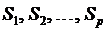on the reel, and denoting by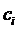the number of symbol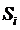on the reel (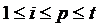), then the vectoris called the distribution of the symbolson the reel, also known as the weighting of a reel. Each reel has its own distribution of symbols. We can assume the same number of distinct symbols on each reel (p) through a convention: if a symbol does not appear on a reel, we could simply take its distribution on that reel as being zero. A blank is considered as a distinct symbol within the mathematical model.

The raw approximation.  This method is based on the well-known result from probability theory called the Bernoulli's Theorem, which states that in a sequence of independent experiments performed under identical conditions, the sequence of the relative frequencies of the occurrence of an event is convergent toward the probability of that event.

Applied to slots, that principle says that if N is the number of spins of a reel with t stops where we observe as an outcome a specific symbol S that is placed on c stops and n(N) is the number of occurrences of S after the N spins, then the sequenceis convergent toward the probability of occurrence of S, namely P(S) = c/t.

The ratio n / N is the relative frequency of occurrence of S.            It follows that for large values of N, the relative frequency of occurrence of S approximates the probability of S occurring. The higher N, the more accurate this approximation. Obviously, the number of spins N must be large enough for obtaining good approximations of the ratios, and this is the main issue of this method. As theory does not provide us with tools for choosing N for a given error range, all we have is the principle “the larger N, the better.”

As one can notice, this method of approximation based on statistical observation is subject to errors coming from idealizations and various assumptions, and the error ranges are not even quantifiable. Note that the described method provides us with approximations of the ratios(usually labeled as "hit frequency" in PAR sheets) for each reel and not the parameters of the configuration individually (and t). However, knowing the basic probabilities is enough for any probability computation for a slot game. A more accurate approximation of the ratiosand even ofand t individually is still possible through statistical observation, using a method which can refine the raw estimations obtained through the previously described method. Such a method is briefly described further.

Denominator-match method.  Denote bythe number of occurrences of symbolsto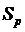respectively after N spins of a reel. There is a slight correlation between the recorded valuesfor various large numbers of spins N. Based upon this correlation, we can refine the estimation of the ratiosobtained through the previous method and also find estimations forand t, by recognizing a numerical pattern across some sequences of fractions representing the ratios between possible values forand t.

The denominator-match method is based on the numerical analysis of the fractionsand on a five-step algorithm, which I describe very briefly in this article and is explained at large in my last book The Mathematics of Slots: Configurations, Combinations, Probabilities:

We write each fractionas a chain of equal fractions, having numerators from 1 upward and denominators not necessarily integers, for every i from 1 to p.  Across the p chains of equal fractions obtained, we choose that of the minimal length (let m be the minimal length). Then, across the p chains of equal fractions, we extract m sequences of fractions (one fraction from each equality chain), having the denominators the nearest to the denominators from the minimal equality chain respectively. From the m sequences of fractions obtained, we choose one sequence of p fractions by applying progressively the following filtering criteria: having denominators as close to each other as possible, having the highest number of instances of the same denominator, and the repeating denominator with the largest share being an integer. As final step, we adjust the numerators of the final sequence of fractions, through an approximation algorithm that leads to one denominator for all the fractions, being the sum of the numerators, as it is the relation between t and numbers.

This method provides us with the most probable number of stops t and associated symbol distributionof a reel in a certain probability field; the error range of this approximation is quantifiable in terms of probability.

Regarding the practical application of the methods through statistical observation, it is obviously an arduous task, since we have to watch and record spins in numbers of thousands.

Any information acquired on t besides the presented statistical methods of estimation is useful with respect to the accuracy of the approximations because it can give a clue as to how high we should choose N for avoiding irrelevant results (for example, if t = 100, we intuit that choosing N = 1,000 or lower would not be high enough for relevant results). Besides the methods based on statistical observation, there exists a method of estimating t through physical measurements, applicable to some particular types of slot machines. This method exploits the information given by the appearance of the reel on the display. As we know, only a small part of the reel (either physical or virtual) is visible on the display and this part can be seen as one or several adjacent stops (usually 3, up to 5). So we can view from 1 up to 5 consecutive stops of the reel. If the appearance of this part of the reel is three-dimensional (which is possible for both physical and virtual reels), by measuring some parameters of this image, we can deduce an estimation for the number of stops of that reel (t). Basically, the apparent lengths of the visible stops give full information on the curvature of the reel, which then leads to an estimation of the entire number of stops, since the number of visible stops per the circular length of the visible reel is proportional to the total number of stops per the circular length of the entire reel. This method can be applied only to reels showing at least two consecutive stops on the display in three-dimensional view. The method cannot be applied to virtual reels showing several consecutive stops in flat image. As in the case of the previous method through statistical observation, there are issues with the practical application of the method through physical measurements. There might be technical issues regarding acquiring the proper position for measurement or placing the measurement tool on the surface of the machine. Also for this method, an alternative would be for the observer to take photos and make the measurements on the photos. Of course, the slot machine operator might not allow the direct measurement and/or taking photos.

An applied mathematics unit using the denominator-match method applied on statistical records for retrieving the missing parametric configurations of the existent slot machines and generating their attached probability results is in project.

With regard to problem gambling, past empirical studies found that facing the odds does not change much gamblers' behavior toward a decrease, however a clear conclusion is not drawn yet. As regard to the slots game itself, the odds and other mathematical facts do count as information in a trivial strategic sense: It is as if someone asks you to bet you can jump from a high place and land on your feet; of course, it is an advantage for you to know in advance the height from which you will jump or measure it before you bet, as you might decline the bet or propose another one for a certain measurement, and this means decision.

Articles index | Gambling probabilitiesSlots index